# Channel results: equilibrium wall-stress model in the Hybrid code

The full results for the equilibrium wall-stress model implemented using the coupling technique described here in a minimally dissipative 6th-order accurate code (the Hybrid code, from JL’s research group) are shown here, for all grids. Results are shown for two different subgrid models, the constant coefficient Vreman model and the dynamic Smagorinsky model (with filtering and averaging in wall-parallel planes).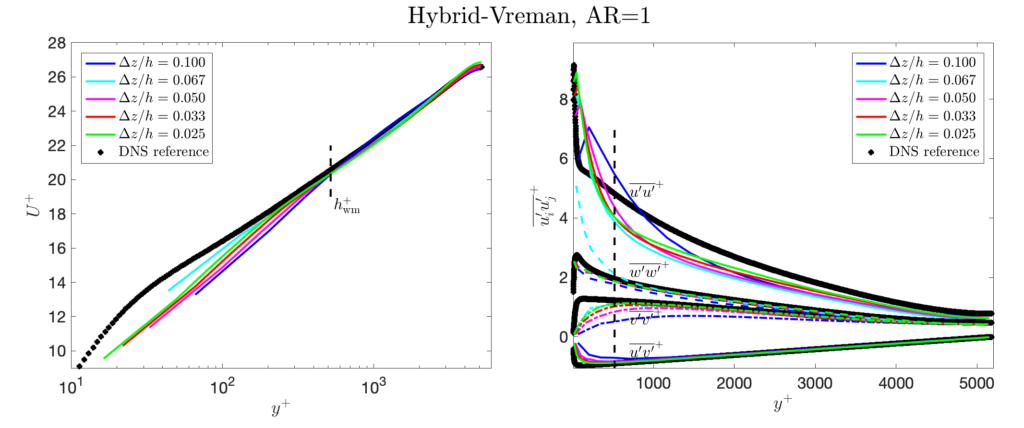The Vreman model with a constant coefficient c=0.07, on grids with AR=1, using the Hybrid code.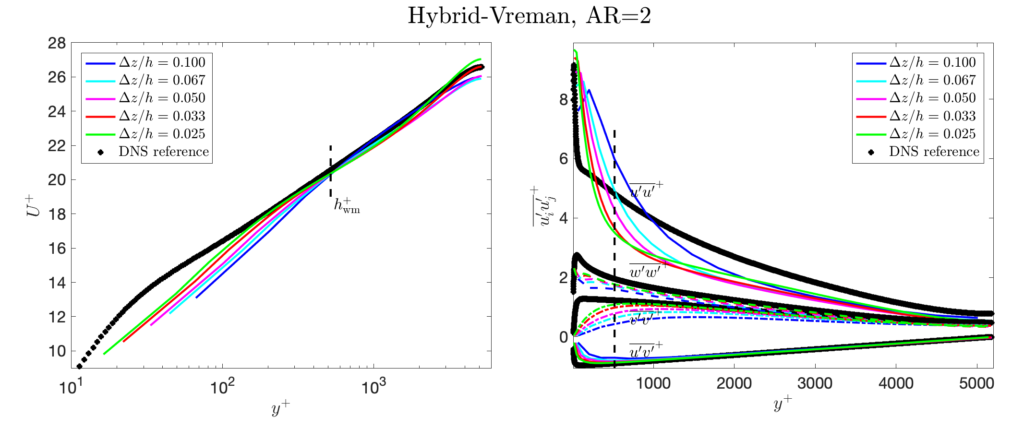The Vreman model with a constant coefficient c=0.07, on grids with AR=2, using the Hybrid code.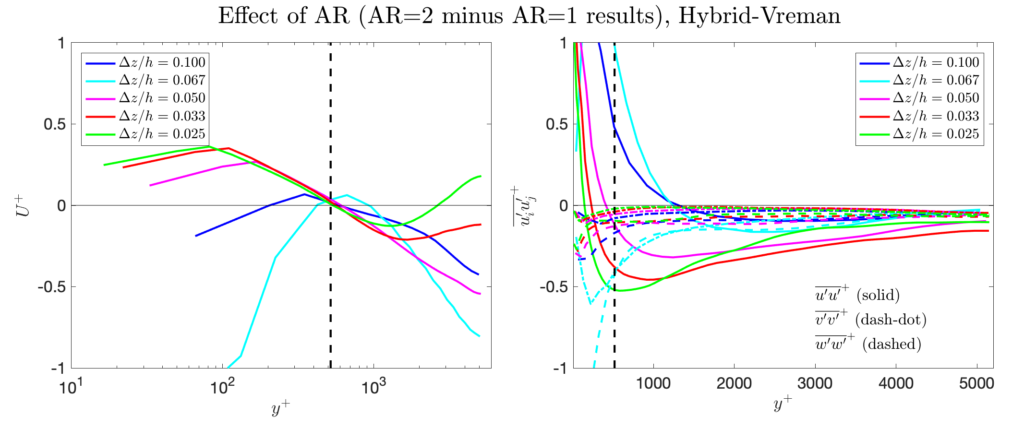The Vreman model with a constant coefficient c=0.07. The difference in the results between the AR=2 and AR=1 grids (where the dy and dz are identical between the grids).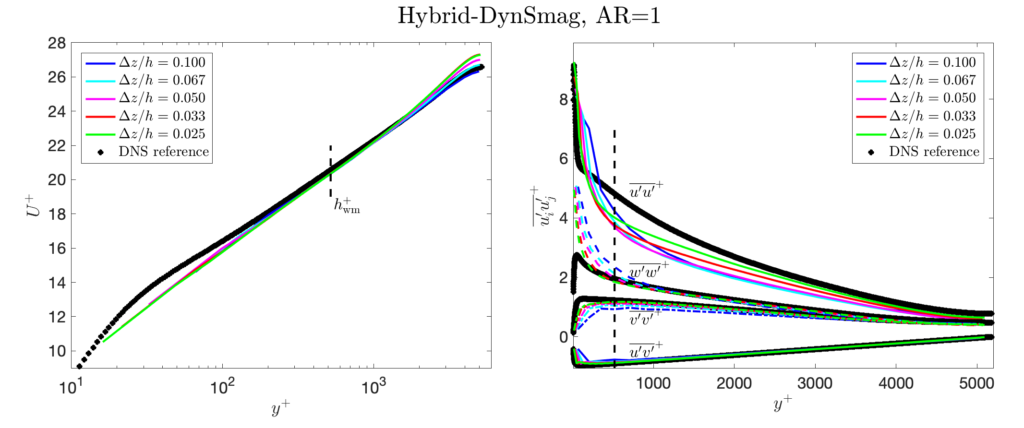The dynamic Smagorinsky model, on grids with AR=1, using the Hybrid code.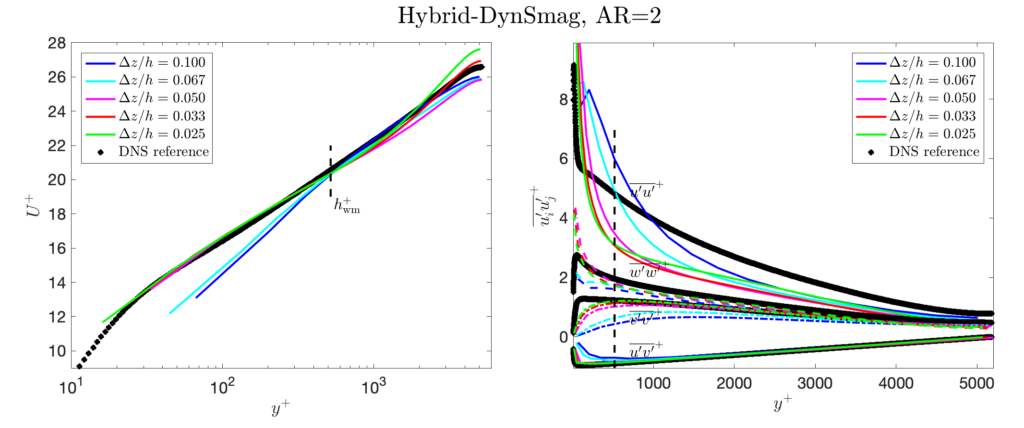The dynamic Smagorinsky model, on grids with AR=2, using the Hybrid code.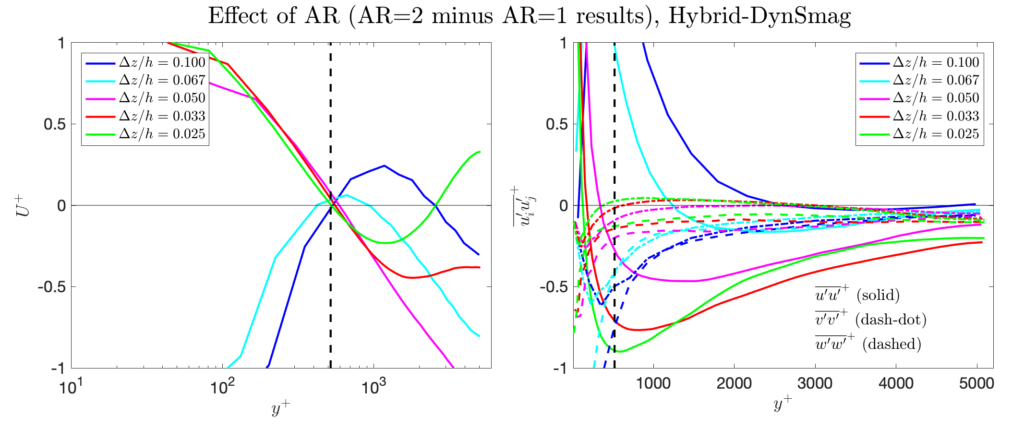The dynamic Smagorinsky model. The difference in the results between the AR=2 and AR=1 grids (where the dy and dz are identical between the grids).